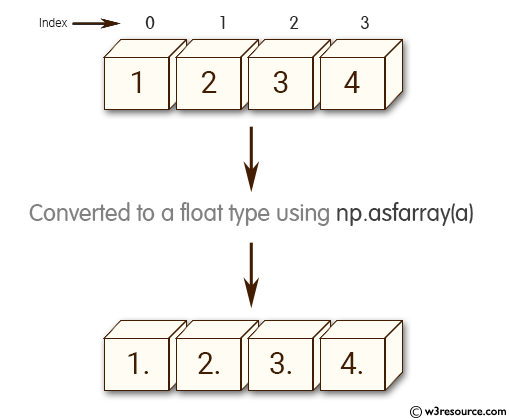﻿ NumPy: Array converted to a float type - w3resource# NumPy: Array converted to a float type

## NumPy: Array Object Exercise-7 with Solution

Write a NumPy program to convert an array to a float type.Sample Solution:-

Python Code:

``````import numpy as np
import numpy as np
a = [1, 2, 3, 4]
print("Original array")
print(a)
x = np.asfarray(a)
print("Array converted to a float type:")
print(x)
```
```

Sample Output:

```Original array
[1, 2, 3, 4]
Array converted to a float type:
[ 1.  2.  3.  4.]
```

Python Code Editor:

Have another way to solve this solution? Contribute your code (and comments) through Disqus.

What is the difficulty level of this exercise?

Test your Python skills with w3resource's quiz

﻿

## Python: Tips of the Day

List comprehension with the current and previous value:

```>>> a = [1, 2, 4,2]
>>> [y - x for x,y in zip(a,a[1:])]
[1, 2, -2]
```